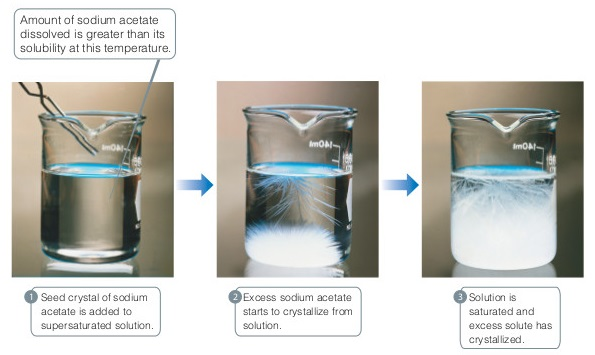# Problem: Precipitation from a supersaturated sodium acetate solution. The solution on the left was formed by dissolving 156 g of the salt in 100 mL of water at 100 oC and then slowly cooling it to 20 oC. Because the solubility of sodium acetate in water at 20 oC is 46 g per 100 mL of water, the solution is supersaturated. Addition of a sodium acetate crystal causes the excess solute to crystallize from solution.How many grams of sodium acetate are in solution in the third beaker?

🤓 Based on our data, we think this question is relevant for Professor Czernuszewicz's class at UH.

###### FREE Expert Solution

In this problem, we are asked to find the grams of sodium acetate that are in solution in the third beaker in the figure above.

A solution is a mixture of two or more substances.

• It can be classified as saturated, unsaturated or supersaturated
• In a Saturated solution, the solvent has reached its maximum amount of dissolved solute.
• In an Unsaturated solution, additional amounts of solute can be further dissolved in the solvent.
• In a Supersaturated solution, the solvent has dissolved beyond its maximum solubility.

Solubility is the ability of a given substance, a solute, to be dissolved in a solvent.

In solutions, there are only two possible reactions of solute to the solvent, that is crystallization and dilution.

• Crystallization is the process of formation of solid crystals from solution.
• Dilution is the process of dissolving (solid) solute into the solvent.

The rate of which is different depending on the type of solution.

• In a saturated solution, the rate of crystallization = the rate of dilution.
• In an unsaturated solution, the rate of crystallization < the rate of dilution.
• In a supersaturated solution, the rate of crystallization > the rate of dilution.

To solve this problem, we must analyze the process of the crystallization of the salt in the solution.###### Problem DetailsPrecipitation from a supersaturated sodium acetate solution. The solution on the left was formed by dissolving 156 g of the salt in 100 mL of water at 100 oC and then slowly cooling it to 20 oC. Because the solubility of sodium acetate in water at 20 oC is 46 g per 100 mL of water, the solution is supersaturated. Addition of a sodium acetate crystal causes the excess solute to crystallize from solution.

How many grams of sodium acetate are in solution in the third beaker?